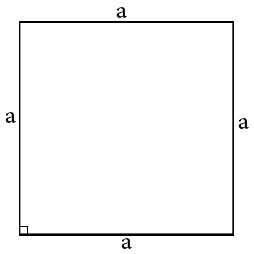# Area of a square calculator.

You could easily calculate square area by our online calculator.Length of leg a:

For square's area calculating it's needed to know it's sides length. After you knew square's side length and keep in mind that all square's sides are equal, you just need to multiply side's length by number of sides or just square it's length. This way we can write formula for square's area:

S = a2,
S=a*a.

### The area of the squareHOME
RESOURCES